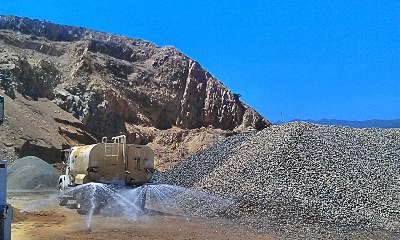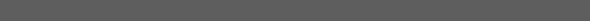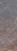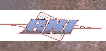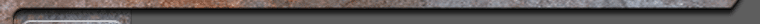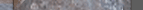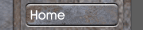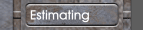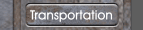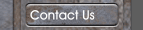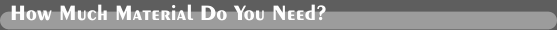All of our aggregate products are sold by weight. Below is a guide on how to figure how much material you will need.Measure the area you need to fill or cover. It is important that all measurements be converted to feet.You will need to get the length, width and depth. Multiply these three measurements to get the total cubic feet.Example:  25.4 x 32 x .34 = 276.36 cubic ftHere is an inch to feet conversion chart to help......1" = .092" = .173" = .254" = .345" = .426" = .507" = .598" = .679" = .7510" = .8411" = .9212" = 1.0Next:Divide the total cubic feet by 27 to come up with the total cubic yards.example: 276.36 ./. 27 = 10.24 cubic yardsFinally:Multiply the total cubic yards by 1.5 to come up with the approximate total number of tons needed.example: 10.24 x 1.5 = 15.36 tons1 Full load on our trucks is approximately 25 tons1/2 Load is approximately 12 1/2 tons